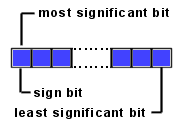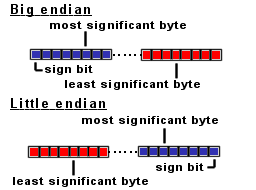# Java Quick Guide

This guide contains useful Java information.

## Java short type.

Information
Short types are so called signed two-complement numbers. The 2 complement scheme allows both positive and negative integers to be represented. The most significant bit of a two complement number is its sign bit. The sign bit is 1 for negative numbers and 0 for positive numbers and for 0.In Java the bytes are big-endian ordered, which means the most significant BYTE (where the sign bit is located) is always on the left side:Short values are stored in 2 bytes and contains positive or negative integer numbers.

The short value range is: 216 = 65536

Half of this number (65536) are positive numbers ranging from: >=0 and <=32767
For example:
0x0000 = 0
0x0001 = 1
0x7FFF = 32767

The other half are negative numbers ranging from: >=-32768 and <0
For example:
0xFFFF = -1
0x8000 = -32768

To convert negative decimal values into hexadecimal values:
value = 216 + (neg_dec_value)
value = 65536 + (neg_dec_value)

For example:
neg_dec_value= -1
value = 65536 + (neg_dec_value) = 65536 + (-1) = 65535
The new decimal value 65535 equals 0xFFFF (Use your Windows calculator, to verify this).

During arithmetic operations the JVM always convert the short value into an int.

 Type Min value Max value Short -32768 32767